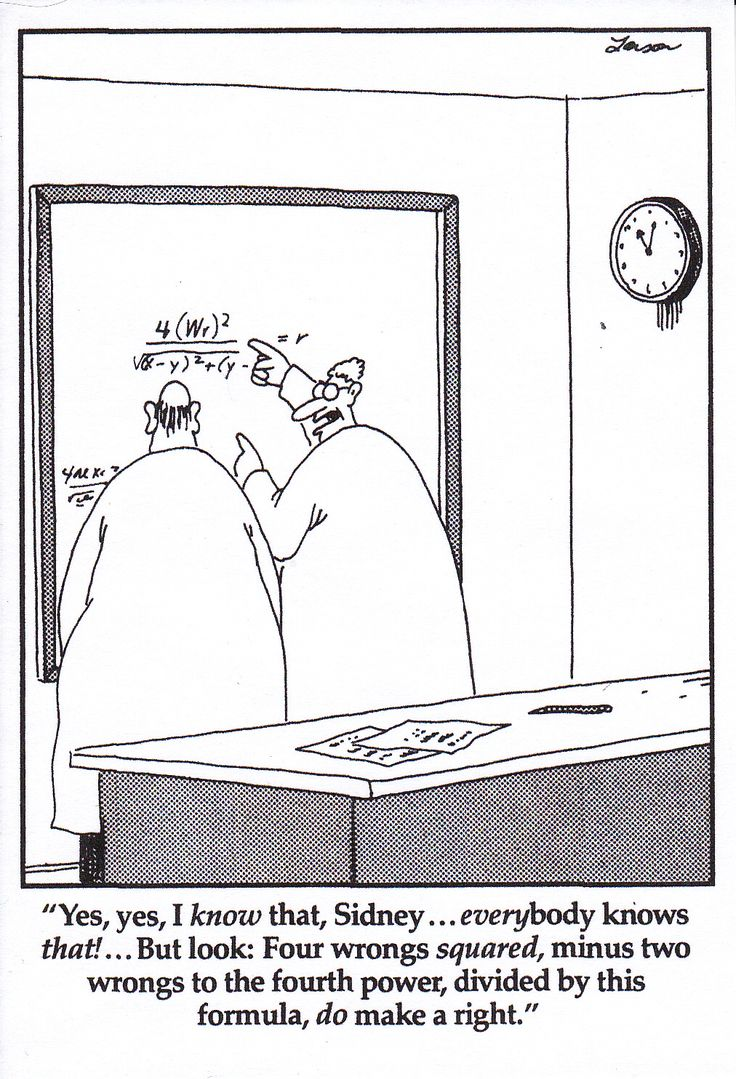# Courses

 I have electronic notes, old exams, and problem sets for each of the courses below. Feel free to contact me for any of these resources. Courses Taught at University of Maryland Spring 2020 MATH 136 : Calculus for Life Sciences Courses Taught at Morehouse College MATH 120 : PreCalculus MATH 130 : Basic Statistics MATH 160 : Calculus for Business MATH 161 : Calculus I MATH 162 : Calculus II MATH 255 : Set Theory MATH 321 : Intro to Ordinary Differential Equations MATH 467 : Numerical Analysis MATH 497 : Senior Seminar Short Courses Advanced Calculus / Real Analysis Introduction to Matlab and dynamical systems What is Data Science?Back to the top